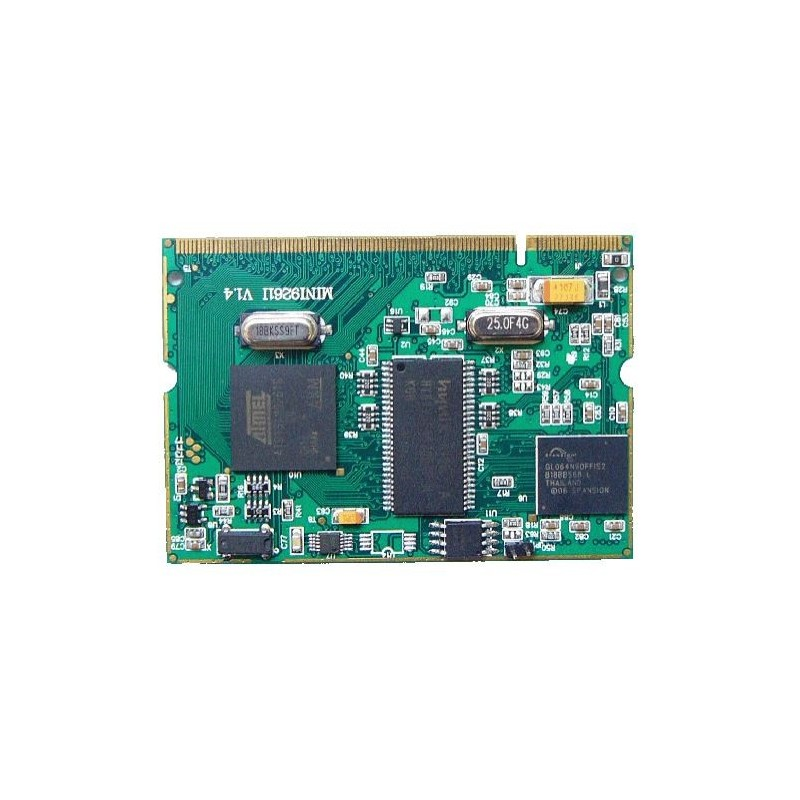﻿
Obniżka!Zobacz większe

# Essential Mathcad for Engineering, Science, and Math ISE

Brent Maxfield

ID: 172185

### Więcej informacji

Using the author's considerable experience of applying Mathcad to engineering problems, Essential Mathcad introduces the most powerful functions and features of the software and teaches how to apply these to create comprehensive calculations for any quantitative subject. The simple, step-by-step approach makes this book an ideal Mathcad text for professional engineers as well as engineering , science, and math students. Examples from a variety of fields demonstrate the power and utility of Mathcad's tools, while also demonstrating how other software, such as Excel spreadsheets, can be incorporated effectively.

*Many more applied examples and exercises from a wide variety of engineering, science, and math fields
* New: more thorough discussions of differential equations, 3D plotting, and curve fitting.
* A step-by-step approach enables easy learning for professionals and students alike

Introduction
Variables
Simple User-Defined Functions
Units!
Templates
Arrays, Vectors, and Matrices